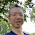## Monday, June 15, 2015

### break-1.0.0: A small library for breaking from loops

I've implemented a small library named `break` for breaking from loops, based off of a previous post of mine.

This library is simple: you wrap the command you want to loop with a function named `loop` and you call `break` when you want to exit from the loop.

``````import Control.Break
import Prelude hiding (break)

example :: State Int ()
example = loop (do
n <- lift get                -- Inside a `loop`, wrap commands in `lift`
if n < 10
then lift (put (n + 1))  -- You keep looping by default
else break () )          -- Use `break` to exit from the `loop```````

The above loop increments `n` until `n` is 10 and then exits from the loop. We can verify this by running the `State` action:

``````>>> execState example 0
10``````

By default, you wrap commands other than `break` with `lift`. However, for some effects (like `State`) you can omit the `lift`:

``````example :: State Int ()
example = loop (do
n <- get
if n < 10
then put (n + 1)
else break () )``````

This library uses a `Break` type which is implemented as `ExceptT` under the hood:

``newtype Break r m a = Break { unBreak :: ExceptT r m a }``

The `break` function just "throws an exception":

``````break :: Monad m => r -> Break r m a
break r = Break (throwE r)``````

Here "throwing an exception" doesn't use any sort of out-of-band feature built into the Haskell language. "Exceptions" are just ordinary values implemented within the language:

``````throwE :: Monad m => e -> ExceptT e m a
throwE = ExceptT (return (Left e))``````

... and `ExceptT`'s implementation of `(>>=)` short-circuits when encountering a `Left` internally, skipping subsequent commands.

`loop` is just an ordinary function that repeats the loop body indefinitely and only stops when you `break` from the loop:

``````loop :: Monad m => Break r m () -> m r
loop m = do
x <- runExceptT (unBreak (forever m))
case x of
Left  r -> return r
Right r -> return r``````

Conceptually we just "catch" the "exceptional value" and return the value. I use quotes because there's no reason to restrict this value to exceptions. You can return any old value from the loop this way:

``````example :: State Int Bool
example = loop (do
n <- get
if n < 10
then put (n + 1)
else break True )

>>> runState example 0
(True,10)``````

Notice how I don't have to define a special variation on the `forever` function that integrates with `ExceptT` or `break` to terminate correctly. Instead, `break` interacts correctly with `forever` out-of-the-box thanks to Haskell's laziness: `forever` only lazily evaluates as many actions as necessary and once the internal `ExceptT` short-circuits the `forever` function doesn't demand any further command repetitions.

This library also showcases one of Haskell's nifty features: the `GeneralizedNewtypeDeriving` language extension. Even though I wrap `ExceptT` in a `Break` newtype, I can still configure `Break` to reuse many of the interfaces that were originally implemented for `ExceptT`, like this:

``````{-# LANGUAGE GeneralizedNewtypeDeriving #-}

newtype Break r m a = Break { unBreak :: ExceptT r m a }
deriving
( Functor
, Applicative
)``````

The `GeneralizedNewtypeDeriving` extension is clever and knows how to wrap and unwrap the newtype to transparently lift all of these type classes to work on `Break`.

In fact, the library implementation is remarkably small. Here's the entire implementation if you omit the documentation:

``````{-# LANGUAGE GeneralizedNewtypeDeriving #-}

import Control.Applicative (Applicative)
import Prelude hiding (break)

newtype Break r m a = Break { unBreak :: ExceptT r m a }
deriving
( Functor
, Applicative
)

break :: Monad m => r -> Break r m a
break r = Break (throwE r)

loop :: Monad m => Break r m () -> m r
loop m = do
x <- runExceptT (unBreak (forever m))
case x of
Left  r -> return r
Right r -> return r``````

Newtypes are probably one of the Haskell features I miss the most when programming in other languages. They are free performance-wise (especially with the recent work on `Data.Coerce`) and you pay very little code to transport interfaces across newtype boundaries. You get all of the benefits of abstraction and precise types at very little cost.

If you would like to use the `break` library you can find the library on Hackage or Github.

1.1.